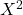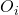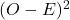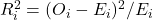Question

The National Highway Traffic Safety Administration reported the percentage of traffic accidents occurring each day of the week. Assume that a sample of accidents provided the following data. Sunday= 64, Monday=53, Tuesday=55, Wednesday=49, Thursday=55, Friday=68, Saturday=76. Conduct a hypothesis test to determine if the proportion of traffic accidents is the same for each day of the week. What is the -value? Compute the value of the test statistic (to 3 decimals).

1.RobertKer

Hence,

a)

Test statistic= 9.269.

p value = 0.148

b)

Sunday=  15.23%, Monday= 12.62%, Tuesday= 13.10%, Wednesday=11.67%,

Thursday= 13.10%, Friday= 16.19%, Saturday= 18.09%.

Step-by-step explanation:

a)

relative observed    Expected       Chi square

Category   frequency(p)Ei=total*p1           0.142857143      64.0         60.00    16.00          0.267

2           0.142857143      53.0       60.00     49.00         0.817

3           0.142857143      55.0         60.00     25.00        0.417

4           0.142857143      49.0       60.00     121.00        2.017

5           0.142857143      55.0         60.00     25.00        0.417

6           0.142857143      68.0         60.00     64.00        1.067

7           0.142857143      76.0         60.00    256.00        4.267

Total         1.000      420        420             556       9.269

Test statistic= 9.269.

p value = 0.148 from excel: chidist(9.269,6)

b)

Percentage %= Frequency of traffic occur on category x 100 / Total number of frequency given.

Category          Percentage%

Sunday                   15.23%

Monday                    12.62%

Tuesday                   13.10%

Wednesday           11.67%

Thursday           13.10%

Friday                   16.19%

Saturday                   18.09%